Hello everybody,

today I want to write a few words about built in data sets in sklearn library of python but from Visual Studio 2017 prospective. You may ask why Visual Studio 2017 if there are plenty of other options? I can say that main reason is becuase I like debugging features that availabile in Visual Studio which make my life much simpler.

So, before we continue, we need as usually in Pythong import some basic libraries. You can do it in Visual studio like this:

```import sklearn
from matplotlib import pylab
import matplotlib
import matplotlib.pyplot as pyplot
import numpy as np

from sklearn import datasets

def sanitize_sequence(data):
return list(data)```

Later you will see why funciton sanitize_sequence is needed.

Data generation

In python you can generate data with following functions:

• make_classification
• make_regression
• make_circles
• make_checkerboard
• and some others

I can't say why those names were chosen, because IMHO make_classification name is better used for something that can classify some data set, but authors of sklearn.dataset decided that it's good idea. So, leave it on their conscience and let's consider some examples of usage. I'd like to mention that those functions allow us to generate data pairs (x, y) which is convenient for drawing on charts.

datasets.make_circles

```circles = datasets.make_circles()
```

This function allows us to generate data set that looks on plane as two circles with one circle inside the other. Consider following code and visualization for it:

```circles = datasets.make_circles()

print ("features: {}".format(circles[:10]))
print( "target: {}".format(circles[:10]))

from matplotlib.colors import ListedColormap

colors = ListedColormap(['red', 'yellow'])

pyplot.figure(figsize=(8, 8))

pyplot.scatter(list(map( lambda x: x, circles)), list(map(lambda x: x, circles)) , c = circles, cmap = colors)

pyplot.show()```

it will give you the following picture: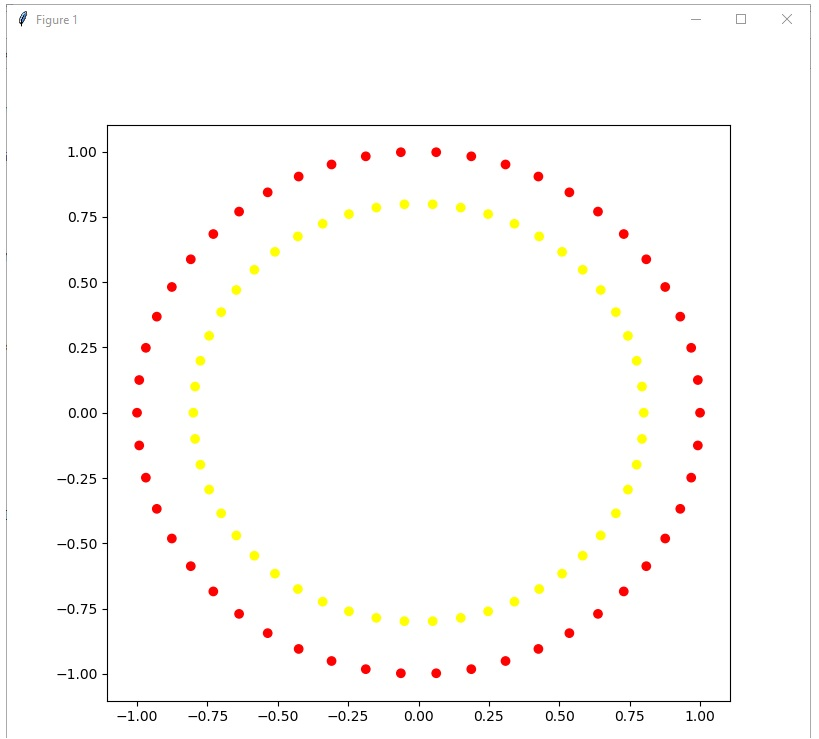Method make circlies gives as output sequence (x, y) or in terms of python tuple that consists of two elements: list of (x,y) coordinates and list of target coordinates.

For the next step let's merge two functions: figure and scatter in one function in order to shorter recordings.

```def plot_2d_dataset(data, colors):
pyplot.figure(figsize(8,8))
pyplot.scatter(list(map( lambda x: x, data)), list(map(lambda x: x, data)) , c = data, cmap = colors)
pyplot.show()```

Having this we can decide to make our life different and generate for ourselves data set with blured borders. Let's try this approach and visualize it with the folloiwng lines of code:

```noisy_circles = datasets.make_circles(noise = 0.05)
plot_2d_dataset(noisy_circles, colors)```

and you'll see following result: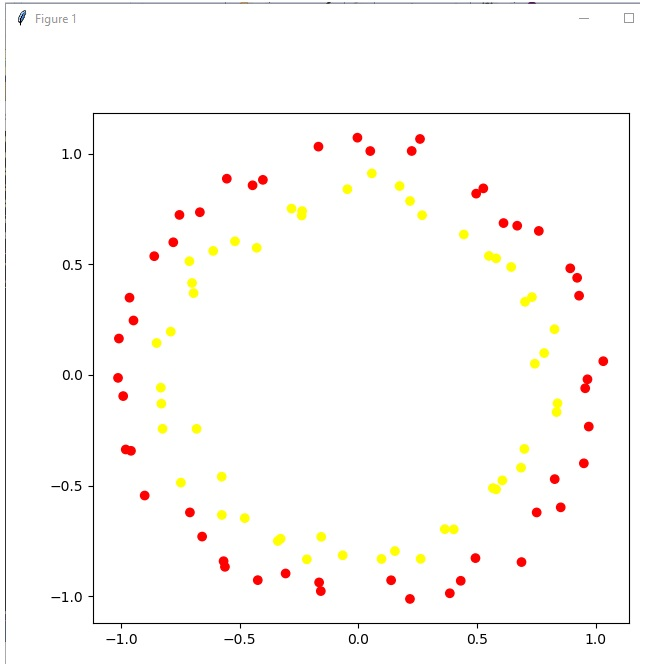as you can see we can use this model for checking power of our model. But let's make model even more blurred: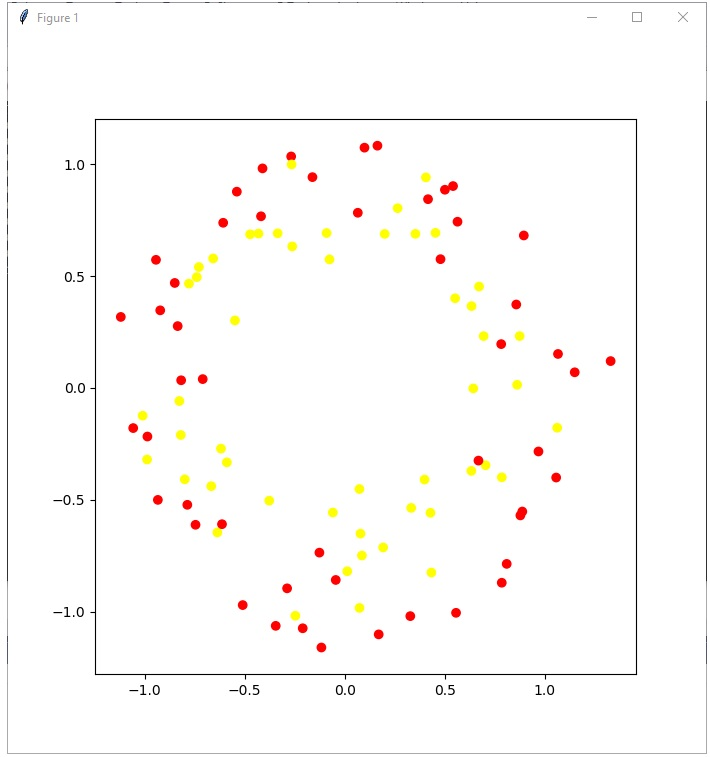with such approach we can see that life for classification model become even more complicated.

Next let's consider function make_classification. Take a look at the code:

```simple_classification_problem = datasets.make_classification(n_features = 2, n_informative = 1,
n_redundant = 1, n_clusters_per_class = 1, random_state = 1)```

With this function we can flexibly generate problem, and we can say how many objects we want to get, quantity of features, which number of features should be informative, and which redundant, we can even add repetative features.

```plot_2d_dataset(simple_classification_problem, colors)
```

Presented code sets numberf of features to 2, which gives us task of binary classification, with 1 informative feature, 1 redundant featue. Take a look how it looks like: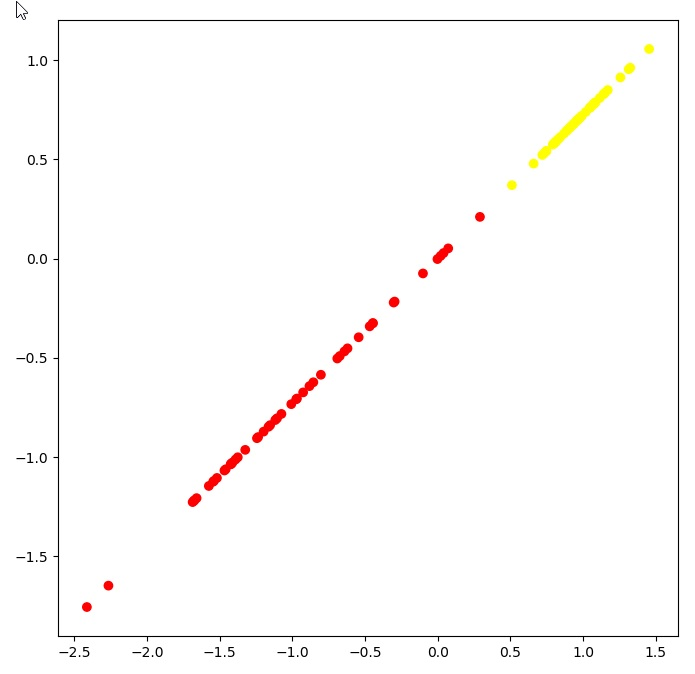As you can see task is very simple, and it's trivial to figure out where to draw a separation line.

Now let's make life harder, and let's add classification for four classes:

```classification_problem = datasets.make_classification(n_features = 2, n_informative = 2,
n_classes = 4, n_redundant = 0, n_clusters_per_class = 1, random_state = 1)
colors = ListedColormap(['red','blue','green','yellow'])
plot_2d_dataset(classification_problem, colors)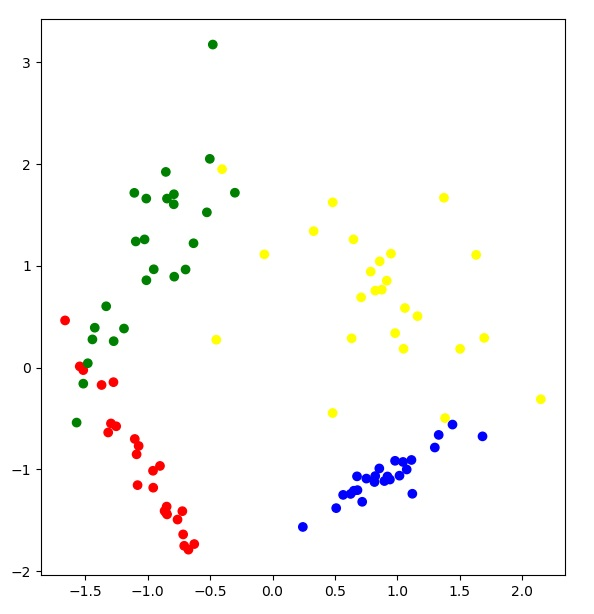```

as you can see from picture we have four different classes of data. With this function you can generate data sets of any level of complexity without need of providing to others production data.

Toy examples

sklearn.datasets has also so called toy examples. You can load them with the following functions:

• etc
```We can take a look how iris looks like:iris = datasets.load_iris()
print(iris)```

and here is screenshot of result: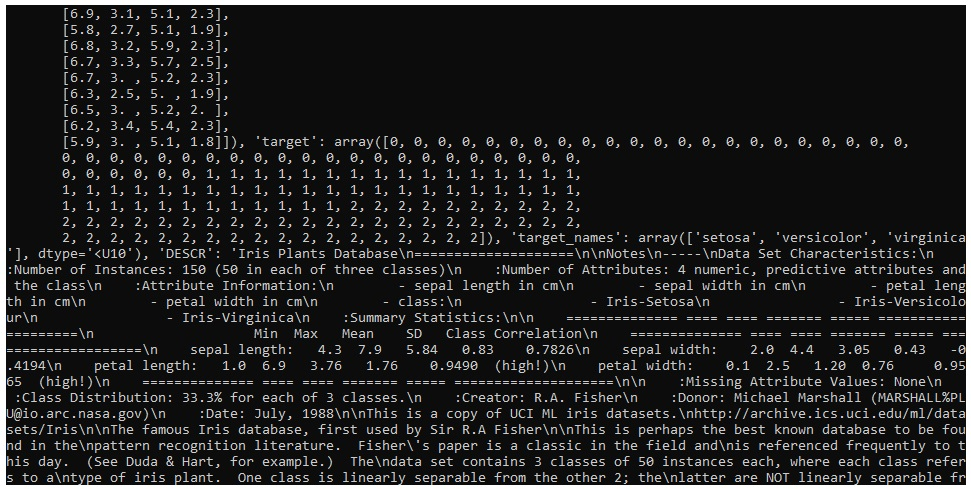as you can see, it looks like iris is some kind of object like dict with some values.

Take a look on keys:

Snippet

`print(iris.keys()) `

and screenshot: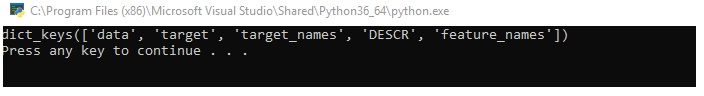Take a look at field DESCR.

I will leave up to you to see how output of DESCR look like, but I can say that it is quite detailed for watching.

Now take a look how data look like. With purpose of simplification of output we will print only first 10 values:

Snippet

`print(iris.data[:10])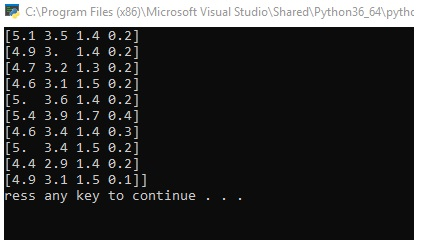`

also take a look at target variable:

print(iris.target)

and here is another output but inside of Visual Studio: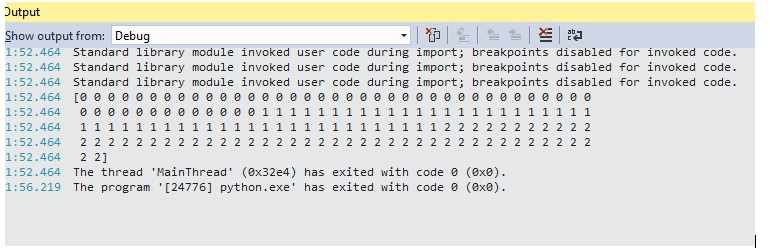so in case if you closed the window, you still will be able to see output.

Visualization of data

Convenience of visualization we will import DataFrame from pandas library. Also take a look at some visualizations. In Visual Studio it can be done like this:

```from pandas import DataFrame

iris_frame = DataFrame(iris.data)
iris_frame.columns = iris.feature_names
iris_frame['target'] = iris.target

In Visual Studio it will give you following visualization: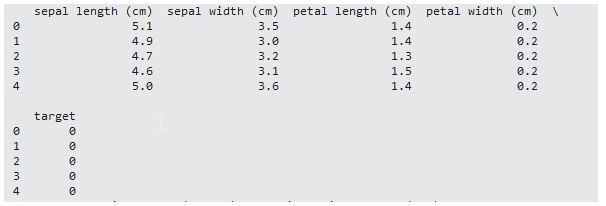I can't say that I like it very much, be we have what we have. In any case, you can see inputs, and also targets for those inputs. If you want to change how the output look like, you can use the following:

```from pandas import DataFrame
import pandas as pd
pd.set_option('display.max_columns', 0)
iris_frame = DataFrame(iris.data)
iris_frame.columns = iris.feature_names
iris_frame['target'] = iris.target

In that case you'll see the following picture: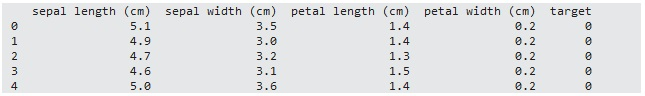IMHO it is more convenient to use then previous multiline view.

For now let's continue with analyzis and replace in column targets numbers with names. This can be done with function apply:

```iris_frame.target = iris_frame.target.apply(lambda x: iris.target_names[x])

take a look at results:

```   sepal length (cm)  sepal width (cm)  petal length (cm)  petal width (cm)  target
0                5.1               3.5                1.4               0.2  setosa
1                4.9               3.0                1.4               0.2  setosa
2                4.7               3.2                1.3               0.2  setosa
3                4.6               3.1                1.5               0.2  setosa
4                5.0               3.6                1.4               0.2  setosa```

Now you can see instead of 0 for target more convenient name like setosa.

Let's check how looks diagram of features for class setosa:

```iris_frame[iris_frame.target == 'setosa'].hist('sepal length (cm)')
pyplot.show()```

and below you can see result of this code: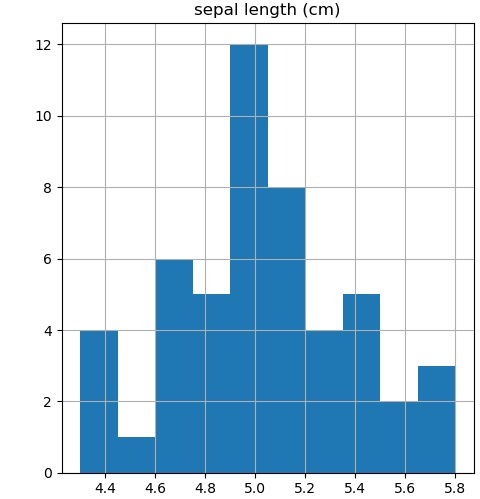What it gives? Well, if you just want to see single distribution, you are done. But in reality it can be needed to analyze multiple distributions. Quite often I can say it is needed to see all features distribution in boundaries of all classes. How to achieve it? Such information will allow us to see are those features differnet, will it become possible to separate somehow those objects. One of the ways to achieve it can be via drawing each feature distribution at a time. But you'll agree that it is not very convenient. Imagine case when you have many features and many classes, that can be cumbersome task. So, what programmers as usually do in such cases? They write cycles. The same is possible in python.

Take a look at following continuation of code:

```plotNumber = 0
for featureName in iris['feature_names']:
for targetName in iris['target_names']:
plotNumber +=1
pyplot.subplot(4,3, plotNumber)
pyplot.hist(iris_frame[iris_frame.target == targetName][featureName])
pyplot.title(targetName)
pyplot.ylabel(featureName[:-4])

pyplot.show()```

It will give you the following output: